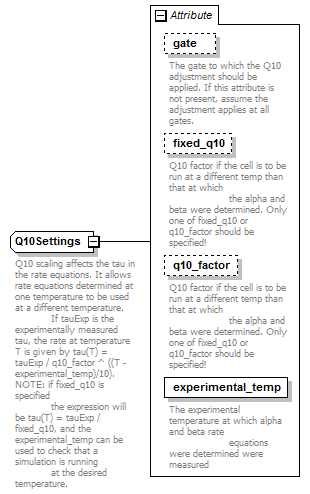# Q10Settings

 ```Q10 scaling affects the tau in the rate equations. It allows rate equations determined at one temperature to be used at a different temperature. If tauExp is the experimentally measured tau, the rate at temperature T is given by tau(T) = tauExp / q10_factor ^ ((T - experimental_temp)/10). NOTE: if fixed_q10 is specified the expression will be tau(T) = tauExp / fixed_q10, and the experimental_temp can be used to check that a simulation is running at the desired temperature.```

## Model## Attributes

QName Type Fixed Default Use Inheritable Annotation
experimental_temp TemperatureValue required
 ```The experimental temperature at which alpha and beta rate equations were determined were measured```
fixed_q10 xs:double optional
 ```Q10 factor if the cell is to be run at a different temp than that at which the alpha and beta were determined. Only one of fixed_q10 or q10_factor should be specified!```
gate xs:string optional
 `The gate to which the Q10 adjustment should be applied. If this attribute is not present, assume the adjustment applies at all gates.`
q10_factor xs:double optional
 ```Q10 factor if the cell is to be run at a different temp than that at which the alpha and beta were determined. Only one of fixed_q10 or q10_factor should be specified!```

## Used By

 ``` Q10 scaling affects the tau in the rate equations. It allows rate equations determined at one temperature to be used at a different temperature. If tauExp is the experimentally measured tau, the rate at temperature T is given by tau(T) = tauExp / q10_factor ^ ((T - experimental_temp)/10). NOTE: if fixed_q10 is specified the expression will be tau(T) = tauExp / fixed_q10, and the experimental_temp can be used to check that a simulation is running at the desired temperature. The gate to which the Q10 adjustment should be applied. If this attribute is not present, assume the adjustment applies at all gates. Q10 factor if the cell is to be run at a different temp than that at which the alpha and beta were determined. Only one of fixed_q10 or q10_factor should be specified! Q10 factor if the cell is to be run at a different temp than that at which the alpha and beta were determined. Only one of fixed_q10 or q10_factor should be specified! The experimental temperature at which alpha and beta rate equations were determined were measured ```